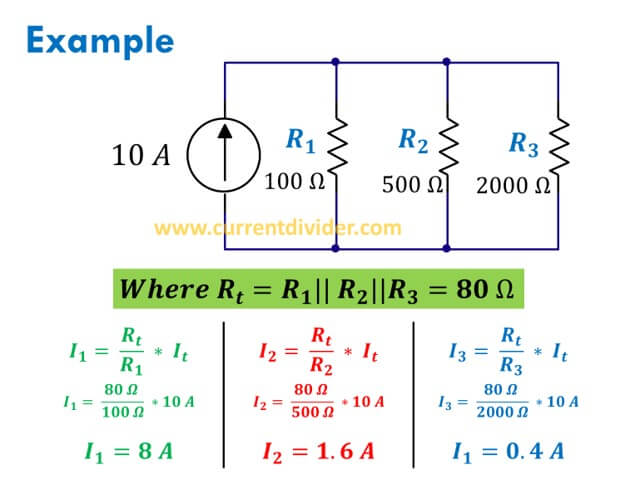# Current Divider

Learn everything about Current Division in Parallel Circuits.

Previously we learned basic formula and statement of current divider rule with various examples. Let's learn the current divider for three parallel resistors.

An electrical circuit has three parallel resistors of 100 ohms, 500 ohms, and 2000 ohms. Apply to the current divider to find the divided current in all resistors when the input source is 10 A.Calculations:
First, three parallel resistors are solved to find the equivalent resistance.

(1/Rt) = (1/R1) + (1/R2) + (1/R3)

(1/Rt) = (1/100) + (1/500) + (1/2000)

1/Rt = 0.01 + 0.002 + 0.0005

1/Rt  = 0.0125

Rt  = 80 Ω

The other formulas are calculated in the figure.